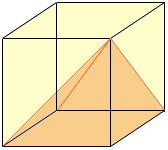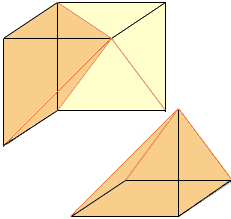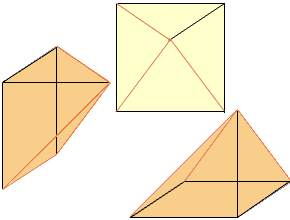Hello there. I come from Singapore. I've got a lot of Mathematics projects this year and there are some questions which I don't understand and need your help. I hope you can help me in any way.

1. What is a hexahedron?(please show a picture of a hexahedron).
2. How do we make a cube out of three pyramids?(show me the picture).
3. How do we find the approximate perimeter and area of a hibiscus leaf?
4. What is a Pascal triangle?

Well, that's for all. If your team could help me, I'd be very grateful. I don't even know how to thank you. I know you have many other questions from other students. But mine are not that difficult, right?I hope you can help me so that I can understand better. Thank you for your co-operation.

Till then, bye.

Hi,

1. A hexahedron is a figure with six faces so, in particular a cube is a hexahedron. So is a rectangular box or even a box that is tilted, as the angles need not be right angles. Also a pyramid on a pentagonal base is a hexahedron.

2. Step 1.Step 2.Step 3.3. I am not sure what you want here. It would depend on how acurate you wanted the approximation and what tools you have to make the approximation.

4. The first five rows of Pascal's triangle are

 1 1 1 1 2 1 1 3 3 1 1 4 6 4 1
Each row begins and ends with a 1 and, after the first two rows, each intervening value is the sum of the two numbers above it. It is easy now to form further rows. If you wish to learn more about Pascal's triangle, search in the Quandaries and Queries database for the word Pascal.

Cheers,
Penny
Go to Math Central